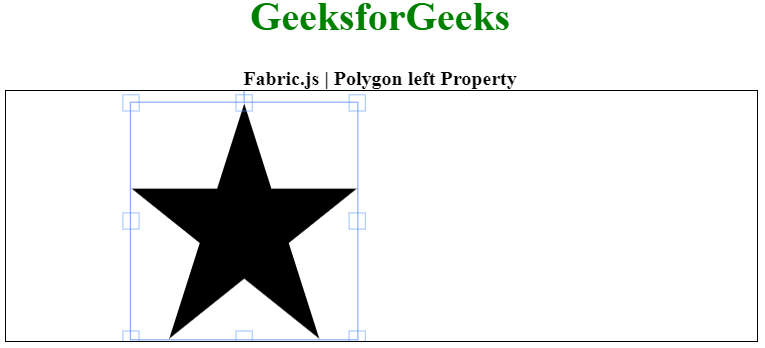Related Articles

# Fabric.js Polygon left Property

• Last Updated : 20 Jan, 2021

In this article, we are going to see how to set the left property of a Polygon canvas using FabricJS. The canvas Polygon means Polygon is movable and can be stretched according to requirement. Further, the Polygon can be customized when it comes to initial stroke color, height, width, fill color, or stroke width.

To make it possible, we are going to use a JavaScript library called FabricJS. After importing the library, we will create a canvas block in the body tag which will contain the Polygon. After this, we will initialize instances of Canvas and Polygon provided by FabricJS and set the value of the left property of a canvas and render the Polygon on the Canvas as given in the below example.

Syntax:

```fabric.Polygon([
{ x: pixel, y: pixel },
{ x: pixel, y: pixel },
{ x: pixel, y: pixel},
{ x: pixel, y: pixel},
{ x: pixel, y: pixel }
],
{
left: number
});```

Parameters: This property accepts a single value as mentioned above and described below:

• left: It specifies the left position of an object.

Note: Dimension pixels is must create a polygon.

Below examples illustrate the Fabric.JS Polygon left in JavaScript:

Example:

## HTML

 ` ``<``html``> `` ` `<``head``> `` ` `    ````    ``<``script` `src``= ``"https://cdnjs.cloudflare.com/ajax/libs/fabric.js/3.6.2/fabric.min.js"``> ``    `` `` `` ` `<``body``> ``    ``<``div` `style``=``"text-align: center;width: 600px;"``> ``        ``<``h1` `style``=``"color: green;"``> ``            ``GeeksforGeeks ``        `` ``        ``<``b``> ``            ``Fabric.js | Polygon left Property ``        `` ``    `` ``    ``<``canvas` `id``=``"canvas"` `width``=``"600"` `height``=``"200"``        ``style``=``"border:1px solid #000000;"``> ``    `` ``     ` `    ``<``script``> ``        ``// Initiate a Canvas instance ``        ``var canvas = new fabric.Canvas("canvas"); `` ` `        ``// Initiate a polygon instance ``        ``var polygon = new fabric.Polygon([ ``            ``{ x: 295, y: 10 }, ``            ``{ x: 235, y: 198 }, ``            ``{ x: 385, y: 78 }, ``            ``{ x: 205, y: 78 }, ``            ``{ x: 355, y: 198 }], { ``             ``left: 100 `` ` `        ``}); `` ` `        ``// Render the polygon in canvas ``        ``canvas.add(polygon); ``    `` `` `` ` ``

Output:Attention reader! Don’t stop learning now. Get hold of all the important Comcompetitivepetitve Programming concepts with the Web Design for Beginners | HTML  course.

My Personal Notes arrow_drop_up